# Mathematics 191 Research Seminar in Mathematical Modeling Lecture

• Slides: 29` Mathematics 191 Research Seminar in Mathematical Modeling Lecture 2 Introduction to Modeling, Part 1 January 24 th, 2005Overview ● What is Modeling? ● Pure Math and Modeling ● The Modeling Process ● Proof in Modeling ● Abstraction / Problem Reduction ● Constraints and Objective Functions ● Case Study: Grade Inflation ● The Wide World of Models ● First Projects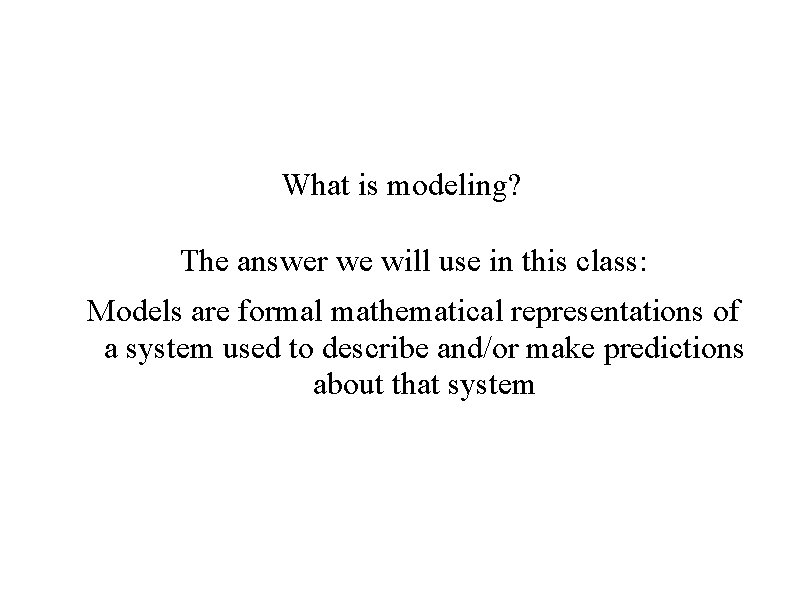What is modeling? The answer we will use in this class: Models are formal mathematical representations of a system used to describe and/or make predictions about that system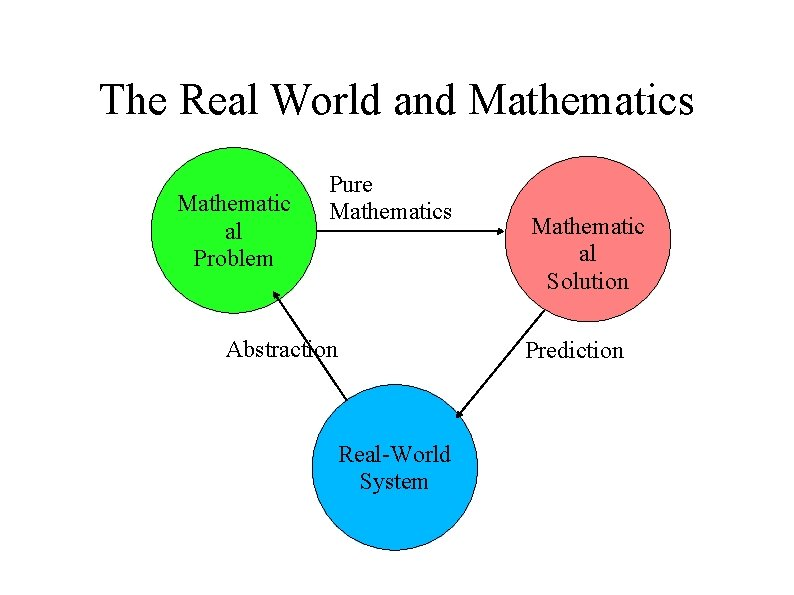The Real World and Mathematics Mathematic al Problem Pure Mathematics Abstraction Mathematic al Solution Prediction Real-World SystemProof in Mathematics vs. Proof in Modeling ● ● As mathematicians, we're used to axioms, theorems, and proofs. Is modeling about proof?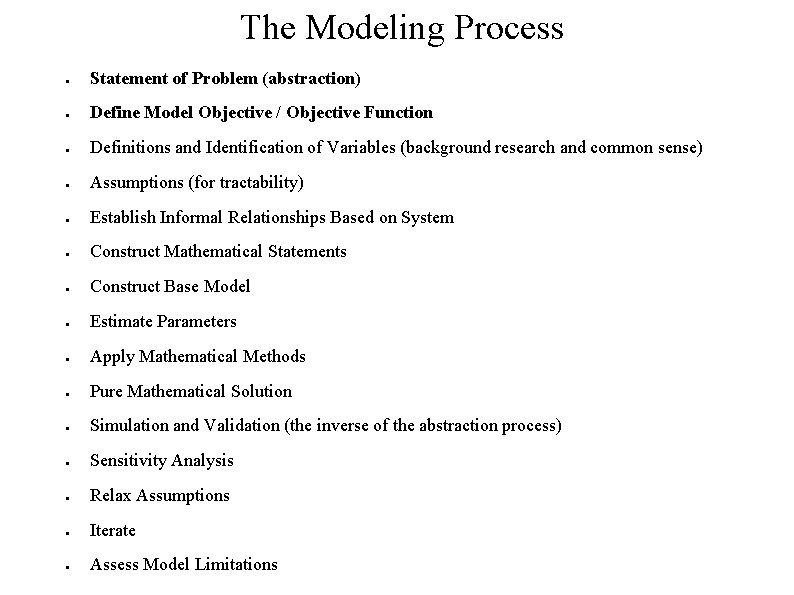The Modeling Process ● Statement of Problem (abstraction) ● Define Model Objective / Objective Function ● Definitions and Identification of Variables (background research and common sense) ● Assumptions (for tractability) ● Establish Informal Relationships Based on System ● Construct Mathematical Statements ● Construct Base Model ● Estimate Parameters ● Apply Mathematical Methods ● Pure Mathematical Solution ● Simulation and Validation (the inverse of the abstraction process) ● Sensitivity Analysis ● Relax Assumptions ● Iterate ● Assess Model Limitations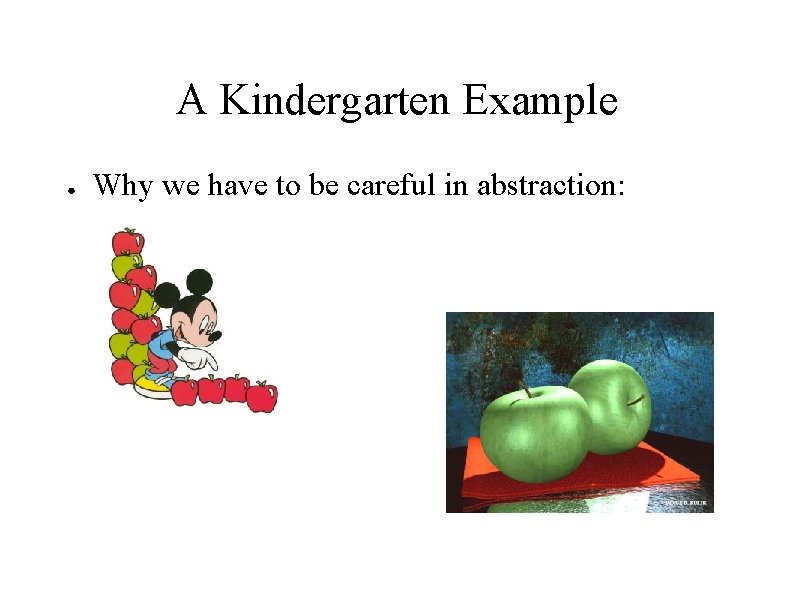A Kindergarten Example ● Why we have to be careful in abstraction: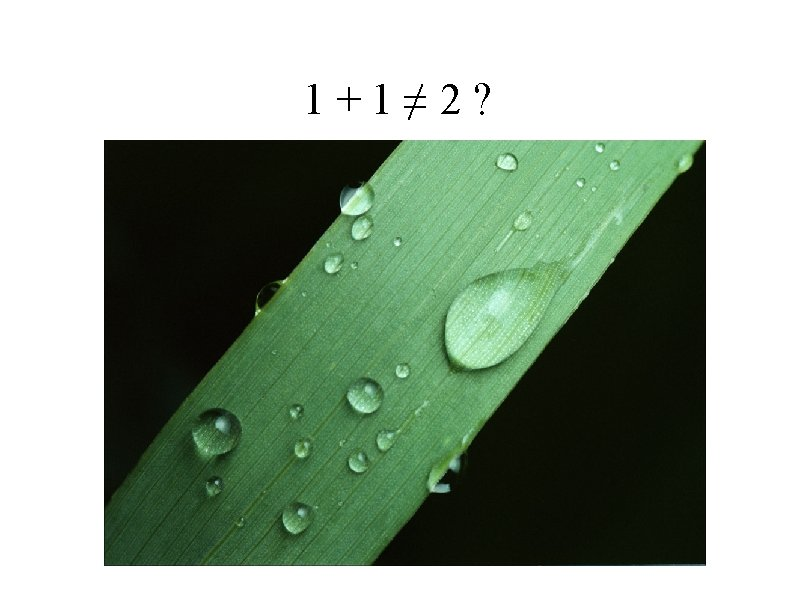Interpretation, Simulation, and Validation Mathematic al Problem Pure Mathematics Abstraction Mathematic al Solution Prediction / Validation Real-World System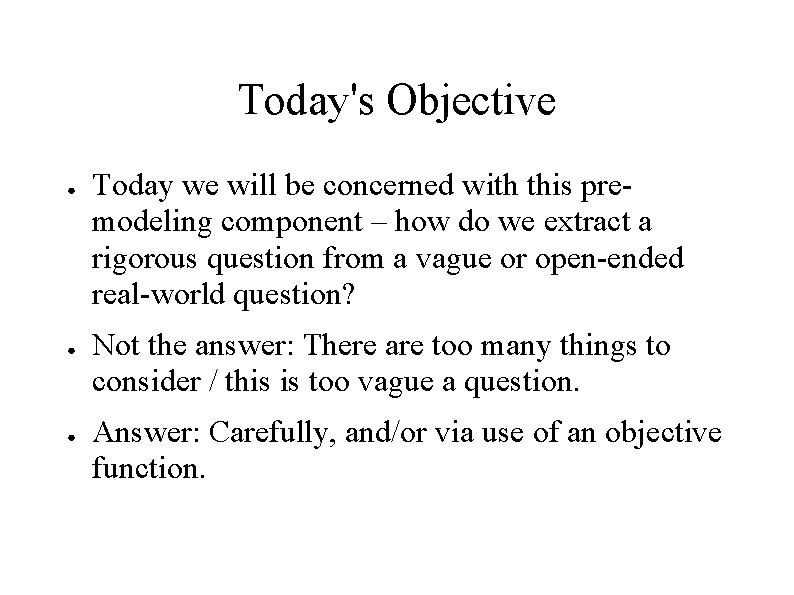Today's Objective ● ● ● Today we will be concerned with this premodeling component – how do we extract a rigorous question from a vague or open-ended real-world question? Not the answer: There are too many things to consider / this is too vague a question. Answer: Carefully, and/or via use of an objective function.A Sample Problem ● ● What is the best way to curb grade inflation? Don't dwell on finding a solution. Simply attempt to construct a rigorous statement of the problem.Statement of Problem ● ● How do we know if our problem is sufficiently rigorous? Are there two or more ways to interpret the question? The question should be stated in such a way that we have removed any doubt about the problem we are going to address This does not reduce the problem to only one solution or model. We have not begun the modeling process yet.Some More Rigorous Questions ● ● What rules can we implement in order to ensure that the average grade across all classes on campus is a C+? How do we alter course evaluation systems to insulate professors from negative consequences of strict grading while accurately evaluating their performance? What grading standards should we implement to ensure conformity between departments on campus? However, these questions still leave open some issues in the problem – what sort?Problem Constraints ● ● These may aid us in reducing the problem to a mathematically rigorous problem, or they make things more difficult. Example: Grade distributions must be equal in every class, in terms of percentage students receiving grades. (What's the problem with this? ) Example: We must be able to rank students sequentially in terms of performance. Example: If A scores higher on all examinations than B, A should receive a higher grade than B. Each of the above approaches is problematic.Model Objective or Goal ● Closely linked to problem statement ● Objective function in optimization problem ● What is our metric for a “good” solution to this problem?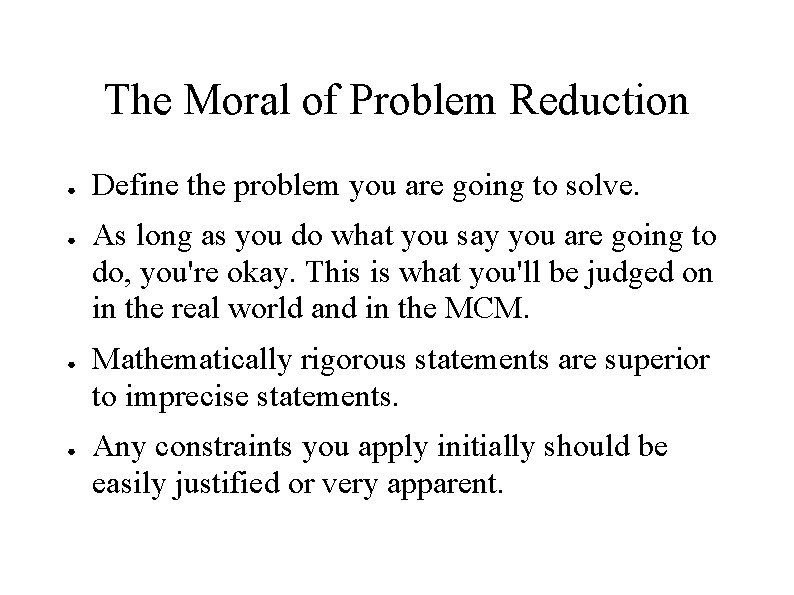The Moral of Problem Reduction ● ● Define the problem you are going to solve. As long as you do what you say you are going to do, you're okay. This is what you'll be judged on in the real world and in the MCM. Mathematically rigorous statements are superior to imprecise statements. Any constraints you apply initially should be easily justified or very apparent.So, to sum it up ● ● ● Modeling constructs formal representations of the relationships in a system in order to describe or predict the system's behavior The abstraction process is concerned with the reduction of open-ended, real-world issues to mathematical questions, first by asking precise questions When beginning the modeling process we should have a clear idea of our goal and objective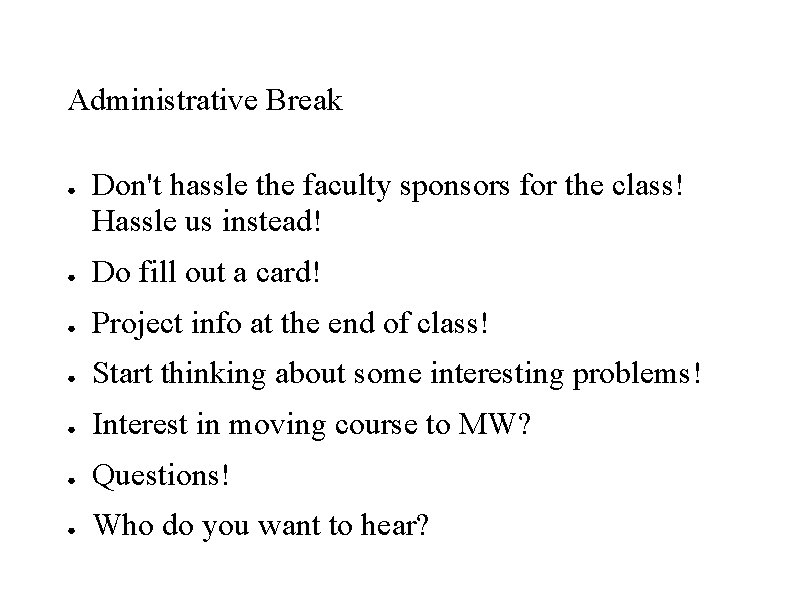Administrative Break ● Don't hassle the faculty sponsors for the class! Hassle us instead! ● Do fill out a card! ● Project info at the end of class! ● Start thinking about some interesting problems! ● Interest in moving course to MW? ● Questions! ● Who do you want to hear?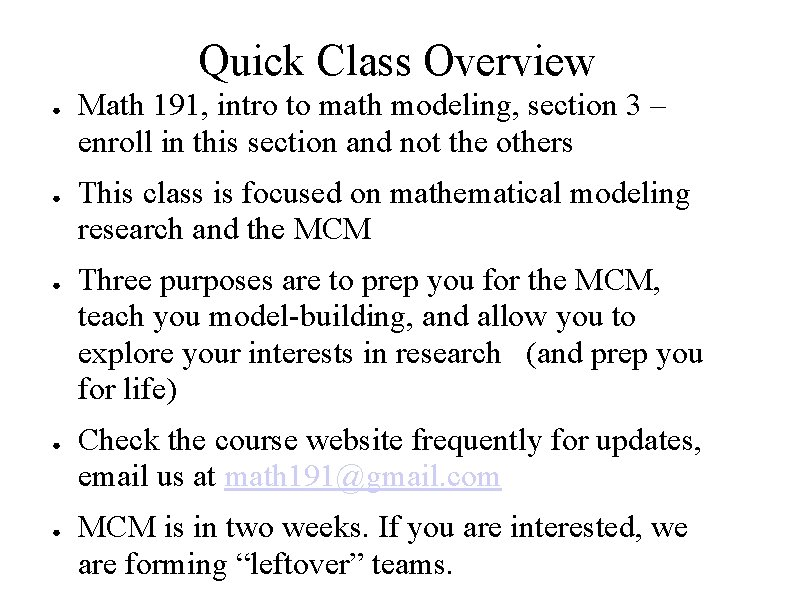Quick Class Overview ● ● ● Math 191, intro to math modeling, section 3 – enroll in this section and not the others This class is focused on mathematical modeling research and the MCM Three purposes are to prep you for the MCM, teach you model-building, and allow you to explore your interests in research (and prep you for life) Check the course website frequently for updates, email us at math [email protected] com MCM is in two weeks. If you are interested, we are forming “leftover” teams.Another open-ended problem ● How should tsunami relief resources be allocated? (Discuss for five minutes – give us an objective function, and a rigorous statement of the problem. Apply some constraints, if desired. ) – Some things I thought about that might be encompassed in a given problem: – Damage distribution geographically – Expected financial resources of nations – What is our objective function? Do we seek to minimize further loss of life, or some other factor? How can we quantify this?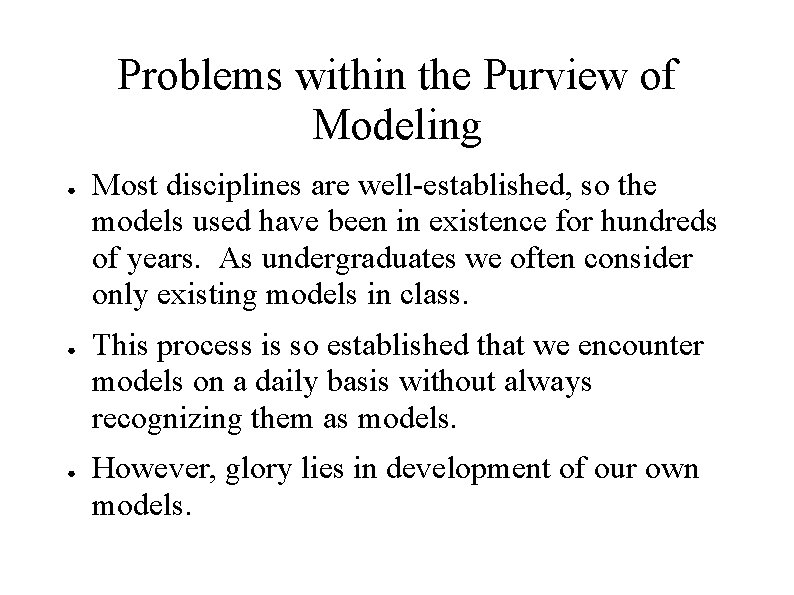Problems within the Purview of Modeling ● ● ● Most disciplines are well-established, so the models used have been in existence for hundreds of years. As undergraduates we often consider only existing models in class. This process is so established that we encounter models on a daily basis without always recognizing them as models. However, glory lies in development of our own models.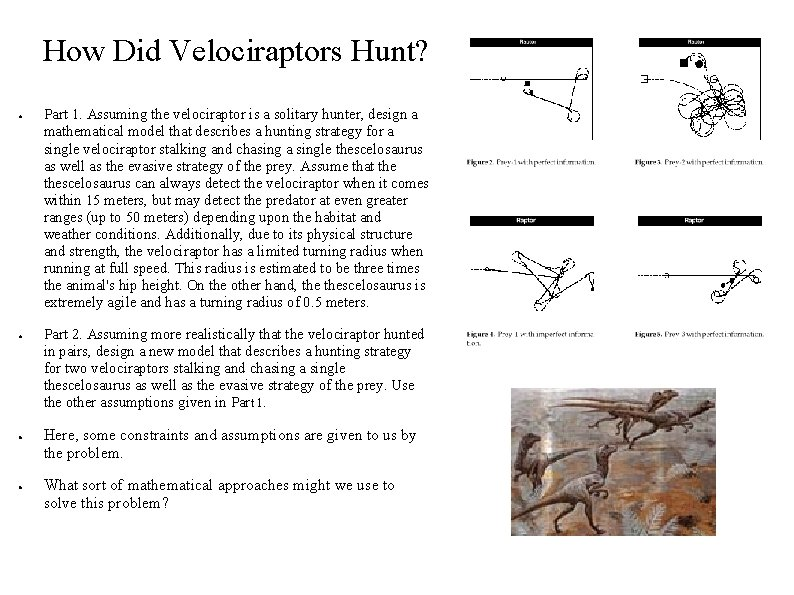How Did Velociraptors Hunt? ● ● Part 1. Assuming the velociraptor is a solitary hunter, design a mathematical model that describes a hunting strategy for a single velociraptor stalking and chasing a single thescelosaurus as well as the evasive strategy of the prey. Assume that thescelosaurus can always detect the velociraptor when it comes within 15 meters, but may detect the predator at even greater ranges (up to 50 meters) depending upon the habitat and weather conditions. Additionally, due to its physical structure and strength, the velociraptor has a limited turning radius when running at full speed. This radius is estimated to be three times the animal's hip height. On the other hand, thescelosaurus is extremely agile and has a turning radius of 0. 5 meters. Part 2. Assuming more realistically that the velociraptor hunted in pairs, design a new model that describes a hunting strategy for two velociraptors stalking and chasing a single thescelosaurus as well as the evasive strategy of the prey. Use the other assumptions given in Part 1. Here, some constraints and assumptions are given to us by the problem. What sort of mathematical approaches might we use to solve this problem?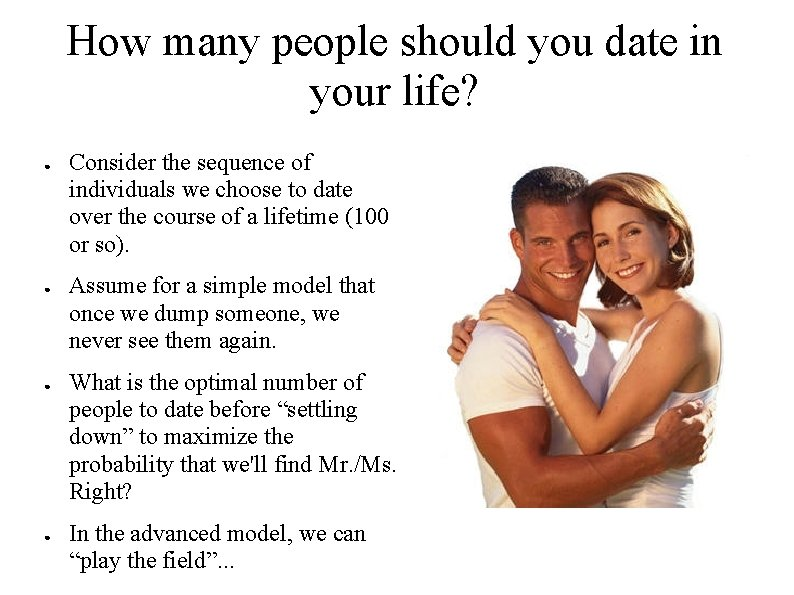How many people should you date in your life? ● ● Consider the sequence of individuals we choose to date over the course of a lifetime (100 or so). Assume for a simple model that once we dump someone, we never see them again. What is the optimal number of people to date before “settling down” to maximize the probability that we'll find Mr. /Ms. Right? In the advanced model, we can “play the field”. . .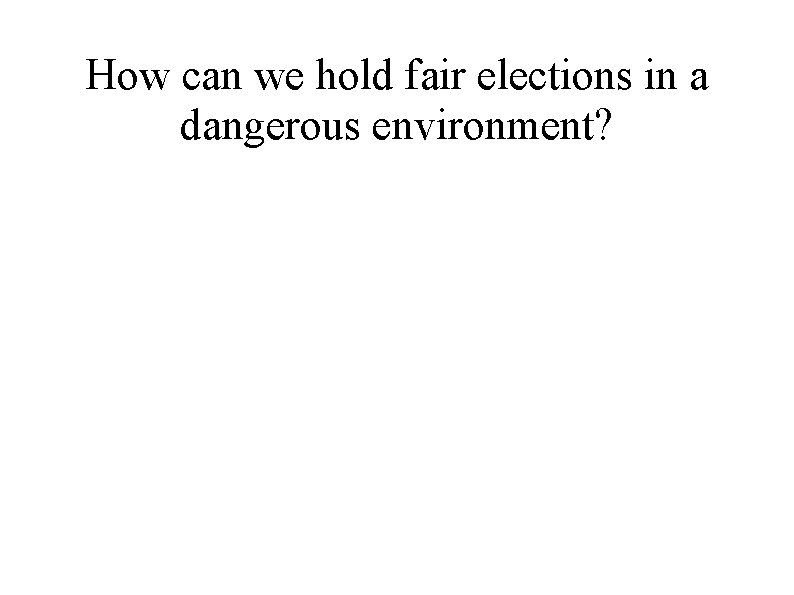How can we hold fair elections in a dangerous environment?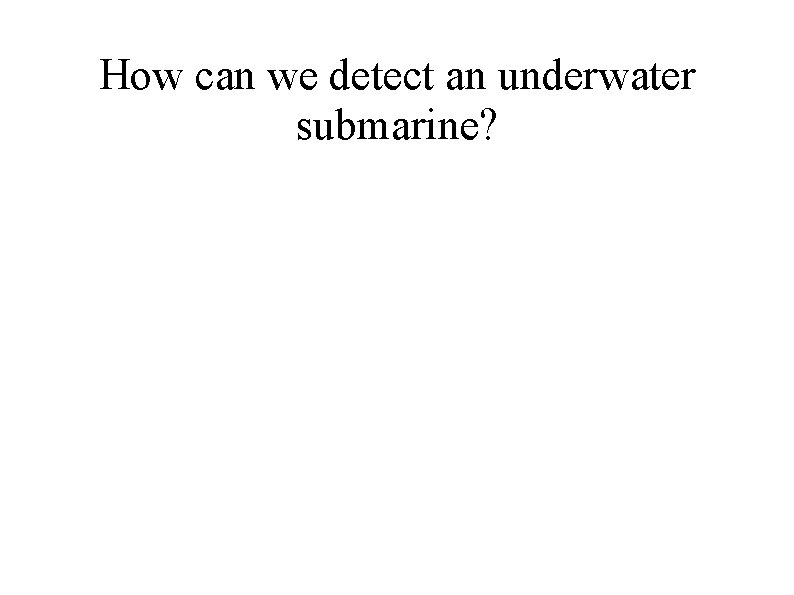How can we detect an underwater submarine?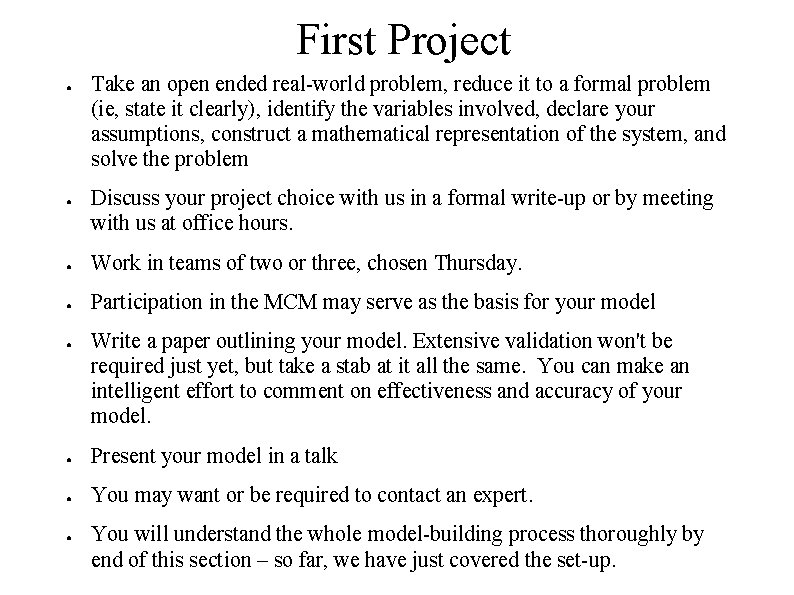First Project ● ● Take an open ended real-world problem, reduce it to a formal problem (ie, state it clearly), identify the variables involved, declare your assumptions, construct a mathematical representation of the system, and solve the problem Discuss your project choice with us in a formal write-up or by meeting with us at office hours. ● Work in teams of two or three, chosen Thursday. ● Participation in the MCM may serve as the basis for your model ● Write a paper outlining your model. Extensive validation won't be required just yet, but take a stab at it all the same. You can make an intelligent effort to comment on effectiveness and accuracy of your model. ● Present your model in a talk ● You may want or be required to contact an expert. ● You will understand the whole model-building process thoroughly by end of this section – so far, we have just covered the set-up.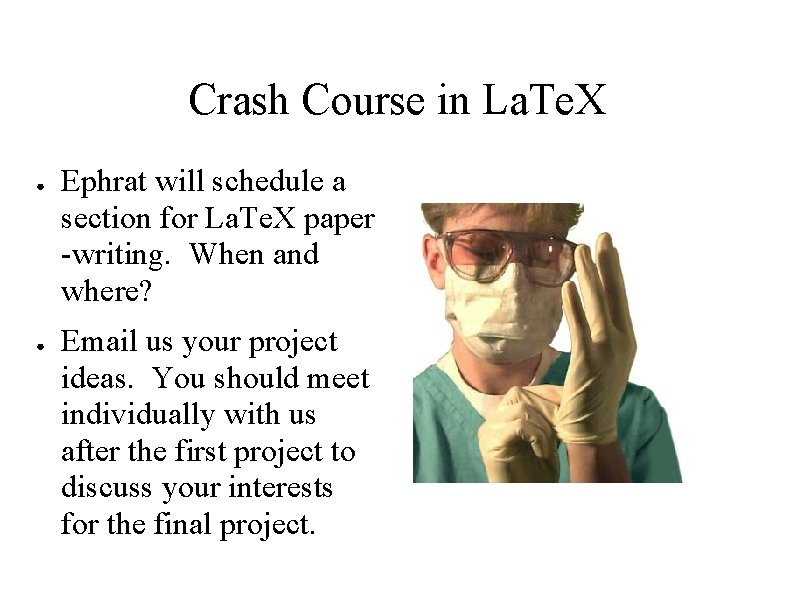Crash Course in La. Te. X ● ● Ephrat will schedule a section for La. Te. X paper -writing. When and where? Email us your project ideas. You should meet individually with us after the first project to discuss your interests for the final project.Fin